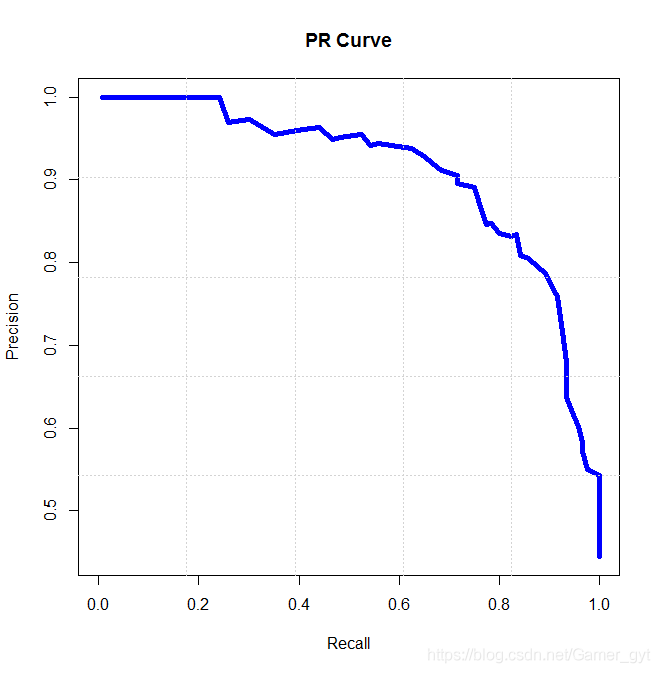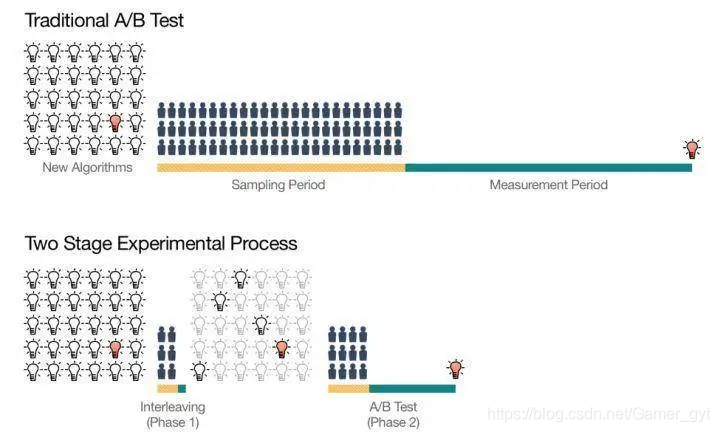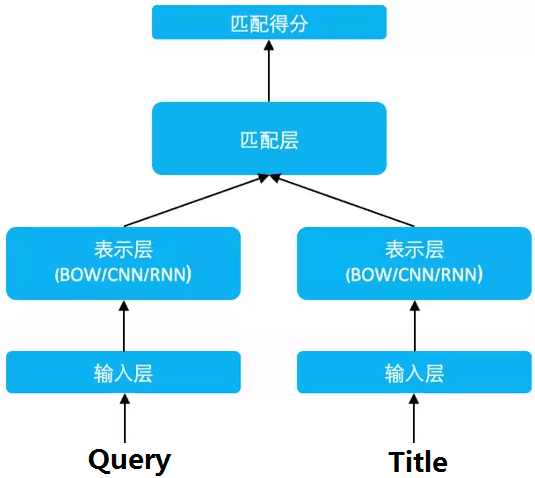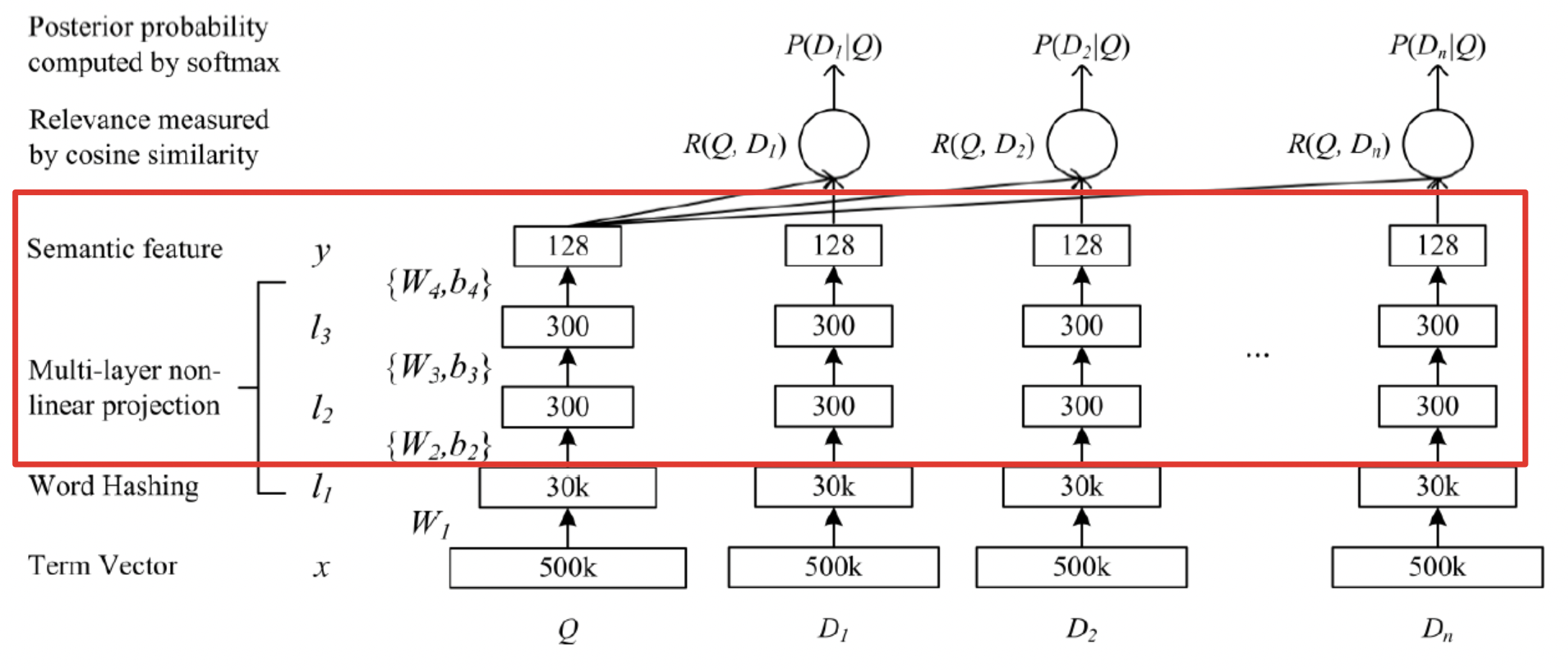## 1. 推荐系统

### 1.1. 推荐系统的产生

1994年明尼苏达大学推出的GroupLens系统一般被认为是个性化推荐系统成为一个相对独立的研究方向的标志。该系统首次提出了基于协同过滤来完成推荐任务的思想，此后，基于该模型的协同过滤推荐引领了个性化推荐系统十几年的发展方向。

### 1.2. 应用场景

#### 1.2.1. 电商领域

• 个性化推荐列表：将那些和用户喜欢的物品比较相似的物品，或者用户好友喜欢的物品推荐给用户。
• 相关推荐列表：当用户购买一件物品后，将那些购买此物品的用户也经常购买的其他物品，或者浏览过此物品的用户也经常购买的其他物品推荐给用户。
• 打包销售：当用户单击某个物品的购买按钮时，将那些其他用户在购买此物品时，连同购买的其他物品推荐给用户。

#### 1.2.2. 电影视频

• Netflix:基于物品的推荐

• Pandora:专家标记
• Last.fm:用户行为

• Foursquare

• Tapestry

### 1.3. 推荐系统的方法

 - 协同过滤推荐（Collaborative Filtering Recommendation）：该方法是应用最广泛的技术之一，需要收集和分析用户的历史行为、活动和偏好。它通常可以分为两个子类：基于用户 （User-Based）的推荐和基于物品（Item-Based）的推荐。该方法的一个关键优势是它不依赖于机器去分析物品的内容特征，因此它无需理解物品本身也能够准确地推荐诸如电影之类的复杂物品；缺点是对于没有任何行为的新用户存在冷启动的问题，同时也存在用户与商品之间的交互数据不够多造成的稀疏问题。值得一提的是，社交网络或地理位置等上下文信息都可以结合到协同过滤中去。

 - 基于内容过滤推荐（Content-based Filtering Recommendation）：该方法利用商品的内容描述，抽象出有意义的特征，通过计算用户的兴趣和商品描述之间的相似度，来给用户做推荐。优点是简单直接，不需要依据其他用户对商品的评价，而是通过商品属性进行商品相似度度量，从而推荐给用户所感兴趣商品的相似商品；缺点是对于没有任何行为的新用户同样存在冷启动的问题。

 - 组合推荐（Hybrid Recommendation）：运用不同的输入和技术共同进行推荐，以弥补各自推荐技术的缺点。

### 1.4. 推荐系统的组成

#### 1.4.1. 画像

 - 定义：画像指的是从用户产生的各种数据中挖掘和抽取用户在不同属性上的标签，如年龄、性别、职业、收入、兴趣等。

 - 画像生成路径

• 用户行为日志收集和存储（离线数据和实时数据）
• 用户行为提取，特征加工，生成特征向量（静态特征和动态特征）
• 利用有用户属性标签的数据作为有标注数据来训练画像预测模型
• 对更多的有标签用户属性来进行预测

 - 画像分类

• 静态画像：用户相对稳定的信息。缺点：实时性不够，过于粗糙
• 动态画像：用户不断变化的行为信息，根据用户行为将物品的结构化结果传递给用户

• 定性画像（定性描述用户或内容的特征信息）
• 定量画像（统计类标签，预测类标签）
• 定性画像+定量验证

 - 画像验证

• 准确率
• 覆盖率

• 在标注数据集里留一部分测试数据用于计算模型的准确率
• 在全量用户中抽一批用户，进行人工标注，评估准确率（数据更可信）
覆盖率指的是被打上标签的用户占全量用户的比例，同理一个覆盖率太低的标签，是没有应用价值的。通常对于覆盖率的评估是以某一个标签覆盖的用户比例和覆盖用户的人均标签数作为评估标准

#### 1.4.2. 召回

 - 定义： 从全量的文章库中按照一定的规则筛选出一个文章候选池，一般的规则有：按照机型，地域，热点和用户-文章协同过滤

 - 召回的作用：从全量内容中，第一次粗过滤，筛选出大概率适合展示给用户的内容，减少后续计算的复杂度

 - 常用召回方法：

#### 1.4.3. 排序

 - 定义： 是推荐系统中召回后的一个模块，主要是一个或多个指标为依据，进行打分，一般将得分按照倒序进行排列

 - 排序的作用

 - 衡量指标

CTR (Click Through Rate)：当给用户推荐他真实喜欢的内容时，用户就会产生比较大的点击意愿，进而产生较高的点击。

## 2. 推荐系统评估指标

### 2.1. 场景转化指标

#### 2.1.1. pv点击率

PV(访问量)：PageView,即页面浏览量或点击量，用户每次刷新即被计算一次。

$$pv点击率=\frac{pv点击}{pv}$$

pv点击率是比较经典的指标，计算用户每次进入页面的情况。他能粗略衡量转化的效果，但是它缺点也比较明显：少数用户贡献大量点击会影响这个指标的作用效果。

#### 2.1.2. uv点击率

UV(独立访客)：Unique Visitor,访问网站的一台电脑客户端为一个访客。00:00-24:00内相同的客户端只会被计算一次。

$$uv点击率=\frac{点击uv}{整个产品的uv}$$

#### 2.1.3. 曝光点击率

$$曝光点击率=\frac{点击量}{曝光次数}$$

#### 2.1.4. uv转化率

$$uv转化率=\frac{点击uv}{进入uv}$$

uv转化率尤其衡量入口型产品，比如视频app的首页，如果用uv点击率去衡量就不太科学，因为一般用户在首页点击某个视频后，会进入下一层页面深度消费，很少会返回首页继续消费，这个用户已经被转化，但不再贡献点击，所以uv转化率更加合理。

#### 2.1.5. 人均点击次数

$$人均点击次数=\frac{点击量}{点击uv}$$

### 2.2. 消费满意度

#### 2.2.1. 留存率

$$留存率=\frac{x日后仍活跃的用户数}{x日前的用户数}$$

#### 2.2.3. 播放完成率

$$播放完成率=\frac{播放时长}{视频时长}$$

### 2.3. 离线评估验证方法

#### 2.3.1. Holdout检验

Holdout检验是基础的离线评估方法，它将原始的样本集合随机划分为训练集和验证集两部分，比如70%训练集，30%测试集（但现在很多机器学习框架、深度学习框架中都增加了验证集，即将整个数据集分成三份，70%训练集，10%验证集，20%测试集）。

Holdout检验的缺点也很明显，即在验证集上计算出来的评估指标与训练集和测试机的划分有直接关系，如果仅进行少量Holdout检验，则得到的结论存在很大的随机性（在划分数据集的时候尽量保证其随机性）。

 - k-fold交叉验证

 - 留一验证

 - 自助法

### 2.4. 离线评估的主要指标

#### 2.4.1. 准确率

$$accuracy=\frac{n_{correct}}{n_{total}}$$

$n_{correct}$为被正确分类的样本个数，$n_{total}$为总样本个数，准确率是分类任务中比较直观的评价指标，但其优缺点也明显。

• 解释性强

• 类别分布不均匀时，占比大的类别往往成为影响准确率的主要因素（极端的情况比如正样本 1%，负样本 99%时）

#### 2.4.2. 精确率和召回率

 - 精确率（Precision）是分类正确的正样本个数占分类器判定为正样本的样本个数的比例

 - 召回率（Recall）是分类正确的正样本个数占真正正样本个数的比例

$$F1=\frac{2*Precision*Recall}{Precision+Recall}$$

#### 2.4.3. 均方根误差

Root Mean Square Error，RMSE 经常被用来衡量回归模型的好坏，使用点击率预估模型构建推荐系统时，推荐系统预测的其实是样本为正样本的概率，RMSE被定义为：

$$RMSE=\sqrt{\frac{1}{n}\sum_{i=1}^n(y_{i}-y_{pred})}$$

$y_{i}$是第i个样本的真实值，$y_{pred}$是第i个样本的预测值，n为样本的个数。

$$MAPE=\sum_{i=1}^n |\frac{y_{i}-y_{pred}}{y_{i}}|*\frac{100}{n}$$

#### 2.4.4. 对数损失函数

LogLoss，在一个二分类问题中，LogLoss定义为：

$$LogLoss=-\frac{1}{N}\sum_{i=1}^N(y_{i}logP_{i}+(1-y_{i})log(1-P_{i}))$$

LogLoss 是逻辑回归的损失函数，大量深度学习模型的输出层是逻辑回归或softmax，因此采用LogLoss作为评估指标能够非常直观的反映模型损失函数的变化，站在模型的角度来讲，LogLoss非常适于观察模型的收敛情况。

#### 2.4.5. P-R曲线

P-R曲线的横轴是召回率，纵轴是精确率，对于一个排序模型来说，其P-R曲线上的一个点代表在某一阈值下，模型将大于该阈值的结果判定为正样本，将小于该阈值的结果判定为负样本时，排序结果对应的召回率和精确率P-R曲线下的面积（Area Under Curve， AUC）能够量化P-R曲线的优劣，AUC越大，排序模型的性能越好。

#### 2.4.6. ROC曲线

ROC曲线的横坐标时 False Posotive Rate（FPR， 假阳性率），纵坐标时True Positive Rate（TPR，真阳性率），FPR和TPR的计算方法如下：

$$FPR=\frac{FP}{N}$$
$$TPR=\frac{TP}{P}$$

ROC曲线的绘制和P-R曲线一样，通过不断移动模型正样本阈值生成的，ROC曲线下的面积就是AUC

#### 2.4.7. 平均精度均值

precision@N1/11/21/32/43/54/6

AP的计算只取正样本处的precision进行平均，即 AP= (1/1 + 2/4 + 3/5 + 4/6) =0.6917

#### 2.4.8.NDCG

 - CG

CG，cumulative，是DCG的前身，只考虑到了相关性的关联程度，没有考虑到位置的因素，它是一个搜索结果相关性分数的综合，指定位置p上的CG为：

$$CG_{p}=\sum_{i=1}^p rel_{i}$$

$rel_{i}$代表i这个位置的相关度。

 - DCG

DCG， Discounted的CG，就是在每一个CG的结果上除以一个折损值，目的是为了让排名越考前的结果越能影响最后的结果，假设排序越靠后，价值越低，那么到第i个位置时，价值为$\frac{1}{log_{2}(i+1)}$，那么第i个结果产生的效益是$rek_{i}*1/log_{2}(i+1)$，所以DCG表达式为：

$$DCG_{p}=\sum_{i=1}^p \frac{rel_{i}}{log_{2}(i+1)}$$
$$=rel_{i}+\sum_{i=2}^{n} \frac{rel_{i}}{log_{2}(i+1)}$$

$$DCG_{p}=\sum_{i=1}^p \frac{2^{rel_{i}}-1}{log_{2}(i+1)}$$

 - NDCG

NDCG，归一化的DCG，由于搜索结果随着检索词的不同，返回的数量是不一样的，而DCG是一个累加的值，没法针对两个不同的检索结果进行归一化出力，这里是除以IDCG。

$$NDCG_{p}=\frac{DCG}{IDCG_{p}}$$

IDCG为理想情况下的最大的DCG的值，为：
$$IDCG_{p}=\sum_{i=1}^{REL} \frac{2^{rel_{i}}-1}{log_{2}(i+1)}$$

#### 2.4.9. 覆盖率

$$覆盖率=\frac{推荐的物品数}{总物品数}$$

#### 2.4.10. 挖掘长尾的能力

1. 所有长尾推荐物品中每天有多少比例被分发出去了
2. 有多少比例的用户，推荐过了长尾物品
3. 长尾内容的转化情况和产生的商业价值

### 2.5. Replay

#### 2.5.1. 动态离线评估方法

Replay方法不仅适用于几乎所有推荐模型的离线评估，而且是强化学习类模型唯一的离线评估方法。

Replay的实际实现中有一点需要特别注意的是：样本中不能包含任何「未来信息」，要避免数据穿越的现象发生

#### 2.5.2. A/B测试与线上评估

A/B测试的分桶原则

• 同层之间进行多组A/B测试，不同测试之间的流量是不重叠的
• 一组A/B测试中实验组和对照组的流量是不重叠的，是互斥的

#### 2.5.3. Interleaving线上评估方法

2013年微软提出了Interleaving线上评估方法，被当作时线上A/B测试的预选阶段进行候选算法的快速筛选，从大量初始想法中筛选出少量优秀的推荐算法，再对缩小的算法集合进行传统的A/B测试，以侧拉他们对用户行为的长期影响。

A/B测试存在的统计学问题

A/B测试虽然是样本进行随机分配，但是难免会存在分布不均匀得情况，我们都知道二八原则，当对平台用户进行分流时，没有办法保证活跃用户也能被均分，因此一种可行的方法就是不对测试人群进行分组，而是让所有测试者都可以自由的选择要测试的物品，在实验结束时，统计每个人选择不同物品的比例，进行相关的数据统计（有点像做选择题哈哈），这种方案的优点在于：Interleaving 方法的优缺点

• 所需样本少
• 测试速度快
• 结果与A/B测试无明显差异

• 工程实现的框架较A/B测试复杂，实验逻辑和业务逻辑纠缠在一起，业务逻辑会被干扰
• Interleaving方法只是对“用户对算法推荐结果偏好程度”的相对测量，不能得出一个算法真实的表现，如果需要知道某个算法的具体指标提升，不适合使用Interleaving

## 3. DSSM

### 3.1. 背景

DSSM（Deep Structured Semantic Models）为计算语义相似度提供了一种思路。

### 3.2. DSSM

DSSM（Deep Structured Semantic Models）的原理很简单，通过搜索引擎里 Query 和 Title 的海量的点击曝光日志，用 DNN 把 Query 和 Title 表达为低纬语义向量，并通过 cosine 距离来计算两个语义向量的距离，最终训练出语义相似度模型。该模型既可以用来预测两个句子的语义相似度，又可以获得某句子的低纬语义向量表达。

DSSM 从下往上可以分为三层结构：输入层、表示层、匹配层#### 3.2.1. 输入层

 - 英文

![word hashing](https://www.aiuai.cn/uploads/paddle/deep_learning/metrics/word_hashing.png)


Letter-Bigram Letter-Trigram
word Size Token Size Collision Token Size Collision
40k 1107 18 10306 2
500k 1607 1192 30621 22

 - 中文

#### 3.2.2. 表示层

DSSM 的表示层采用 BOW（Bag of words）的方式，相当于把字向量的位置信息抛弃了，整个句子里的词都放在一个袋子里了，不分先后顺序。$$l_{1}=W_{1}x$$
$$l_{i}=f(W_{i}l_{i-1}+b_{i}) ,i=2,...,N-1$$
$$y=f(W_{N}l_{N-1}+b_{N})$$

$$f(x)=\frac{1-e^{-2x}}{1+e^{-2x}}$$

#### 3.2.3. 匹配层

Query 和 Doc 的语义相似性可以用这两个语义向量(128 维) 的 cosine 距离来表示：

$$R(Q,D)=cosine(y_{Q},y_{D})=\frac{y_{Q}^Ty_{D}}{||y_{Q}|| ||y_{D}||}$$

$$P(D^{+}|Q)=\frac{exp(\gamma R(Q,D^{+}))}{\sum_{D^{'}\in D}exp(\gamma R(Q,D^{'}))}$$

$$L(\Lambda)=-log \prod_{(Q,D^{+})}P(D^{+}|Q)$$

### 3.3. 参考文献

. Huang P S, He X, Gao J, et al. Learning deep structured semantic models for web search using clickthrough data[C]// ACM International Conference on Conference on Information & Knowledge Management. ACM, 2013:2333-2338.

Last modification：September 18th, 2021 at 11:22 pm